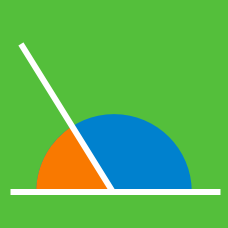Geometry

# Types of Angles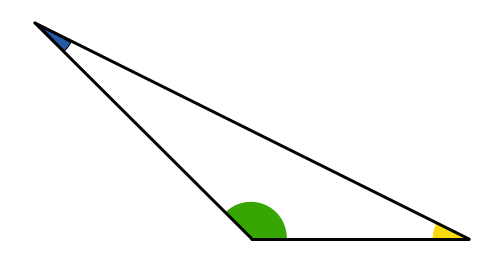How many acute angles does an obtuse triangle have?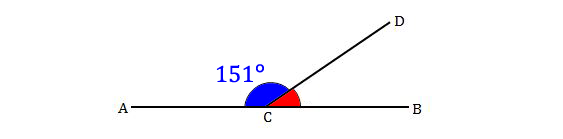In the above diagram, $A,$ $B,$ and $C$ lie on a straight line. If $\color{#3D99F6}{ \angle ACD ={151}^\circ},$ which of the following is true of $\color{#D61F06} { \angle BCD}$?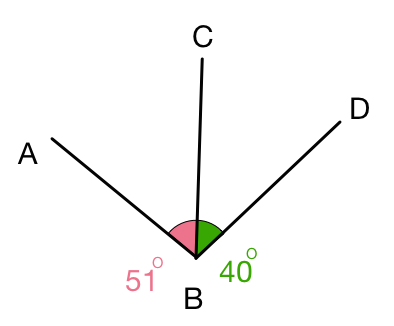Angles $ABC$ and $CBD$ are adjacent with common vertex $B$ and common edge $\overline{BC}.$ If $\angle ABC={51}^\circ$ and $\angle CBD={40}^\circ,$ which of the following is true of $\angle ABD?$

If $\angle A$ is an acute angle, $\angle B$ is a right angle and $\angle C$ is an obtuse angle, which of the following is true of $\angle A+\angle B+\angle C ?$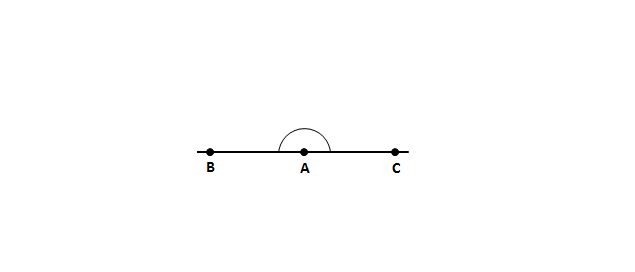In the above diagram, $A$, $B$, and $C$ lie on a straight line. Which of the following is true of angle $\angle BAC$?

×